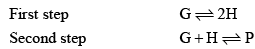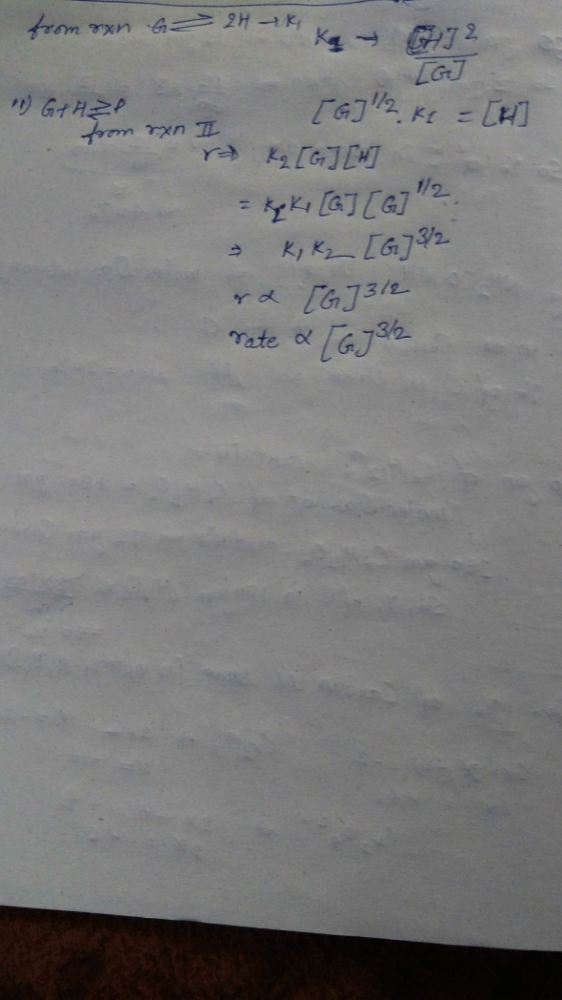Courses

# For a reaction involving two steps given below:Assume that the first step attains equilibrium rapidly. The rate of formation of P is proportional to a)[G]1/2b)[G]c)[G]2d)[G]3/2Correct answer is option 'D'. Can you explain this answer? Related Test: Test: Chemical Kinetics - 2

## Chemistry QuestionRising Star Feb 04, 2019This discussion on For a reaction involving two steps given below:Assume that the first step attains equilibrium rapidly. The rate of formation of P is proportional toa)[G]1/2b)[G]c)[G]2d)[G]3/2Correct answer is option 'D'. Can you explain this answer? is done on EduRev Study Group by Chemistry Students. The Questions and Answers of For a reaction involving two steps given below:Assume that the first step attains equilibrium rapidly. The rate of formation of P is proportional toa)[G]1/2b)[G]c)[G]2d)[G]3/2Correct answer is option 'D'. Can you explain this answer? are solved by group of students and teacher of Chemistry, which is also the largest student community of Chemistry. If the answer is not available please wait for a while and a community member will probably answer this soon. You can study other questions, MCQs, videos and tests for Chemistry on EduRev and even discuss your questions like For a reaction involving two steps given below:Assume that the first step attains equilibrium rapidly. The rate of formation of P is proportional toa)[G]1/2b)[G]c)[G]2d)[G]3/2Correct answer is option 'D'. Can you explain this answer? over here on EduRev! Apart from being the largest Chemistry community, EduRev has the largest solved Question bank for Chemistry.
This discussion on For a reaction involving two steps given below:Assume that the first step attains equilibrium rapidly. The rate of formation of P is proportional toa)[G]1/2b)[G]c)[G]2d)[G]3/2Correct answer is option 'D'. Can you explain this answer? is done on EduRev Study Group by Chemistry Students. The Questions and Answers of For a reaction involving two steps given below:Assume that the first step attains equilibrium rapidly. The rate of formation of P is proportional toa)[G]1/2b)[G]c)[G]2d)[G]3/2Correct answer is option 'D'. Can you explain this answer? are solved by group of students and teacher of Chemistry, which is also the largest student community of Chemistry. If the answer is not available please wait for a while and a community member will probably answer this soon. You can study other questions, MCQs, videos and tests for Chemistry on EduRev and even discuss your questions like For a reaction involving two steps given below:Assume that the first step attains equilibrium rapidly. The rate of formation of P is proportional toa)[G]1/2b)[G]c)[G]2d)[G]3/2Correct answer is option 'D'. Can you explain this answer? over here on EduRev! Apart from being the largest Chemistry community, EduRev has the largest solved Question bank for Chemistry.